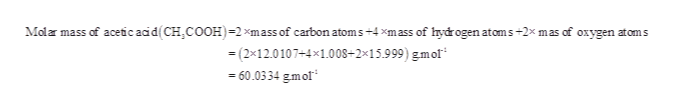# Acetic acid (HC2H3O2) is the active ingredient in vinegar. Calculate the mass percent composition of carbon in acetic acid.Express the mass percentage to four significant figures.

Question
407 views
Acetic acid (HC2H3O2) is the active ingredient in vinegar. Calculate the mass percent composition of carbon in acetic acid.
Express the mass percentage to four significant figures.
check_circle

Step 1

The way of representation of concentration of an element in a compound or a component of a mixture is known as mass percentage.

Step 2

The mass percentage can be calculated as follows:

Step 3

Since, mass of acetic acid and vinegar is not given so molar mass is used in calculating mass percent of the component.

Molar mass of a substan...help_outlineImage TranscriptioncloseMolar mass of acetic acid (CH,COOH)=2xmassof carbon atoms+4xmass of hydrogen atoms+2x mas of oxygen atom s (2x12.0107+4x100os+2x15999) gmol 60.0334 gmol fullscreen

### Want to see the full answer?

See Solution

#### Want to see this answer and more?

Solutions are written by subject experts who are available 24/7. Questions are typically answered within 1 hour.*

See Solution
*Response times may vary by subject and question.
Tagged in

### General Chemistry